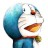# 杰拉斯的博客

## [ACM_HDU_1515]Anagrams by Stack杰拉斯 | 时间：2012-05-09, Wed | 7,778 views

### Anagrams by Stack

Time Limit: 2000/1000 MS (Java/Others) Memory Limit: 65536/32768 K (Java/Others)
Total Submission(s): 614 Accepted Submission(s): 311

#### Description

How can anagrams result from sequences of stack operations? There are two sequences of stack operators which can convert TROT to TORT:

```[
i i i i o o o o
i o i i o o i o
]
```

where i stands for Push and o stands for Pop. Your program should, given pairs of words produce sequences of stack operations which convert the first word to the second.

A stack is a data storage and retrieval structure permitting two operations:

Push - to insert an item and
Pop - to retrieve the most recently pushed item
We will use the symbol i (in) for push and o (out) for pop operations for an initially empty stack of characters. Given an input word, some sequences of push and pop operations are valid in that every character of the word is both pushed and popped, and furthermore, no attempt is ever made to pop the empty stack. For example, if the word FOO is input, then the sequence:

i i o i o o is valid, but
i i o is not (it's too short), neither is
i i o o o i (there's an illegal pop of an empty stack)

Valid sequences yield rearrangements of the letters in an input word. For example, the input word FOO and the sequence i i o i o o produce the anagram OOF. So also would the sequence i i i o o o. You are to write a program to input pairs of words and output all the valid sequences of i and o which will produce the second member of each pair from the first.

#### Input

The input will consist of several lines of input. The first line of each pair of input lines is to be considered as a source word (which does not include the end-of-line character). The second line (again, not including the end-of-line character) of each pair is a target word. The end of input is marked by end of file.

#### Output

For each input pair, your program should produce a sorted list of valid sequences of i and o which produce the target word from the source word. Each list should be delimited by

```[
]
```

and the sequences should be printed in "dictionary order". Within each sequence, each i and o is followed by a single space and each sequence is terminated by a new line.

```
bahama
bahama
long
short
eric
rice
```

#### Sample Output

```
[
i i i i o o o i o o
i i i i o o o o i o
i i o i o i o i o o
i i o i o i o o i o
]
[
i o i i i o o i i o o o
i o i i i o o o i o i o
i o i o i o i i i o o o
i o i o i o i o i o i o
]
[
]
[
i i o i o i o o
]
```

#### Source

```#include <iostream>
#include <string>
#include <iterator>
#include <vector>
#include <algorithm>

using namespace std;

void Solve(string wordA, string wordB, vector<string> &solutions, string resultA = "", string resultB = "", string solution = ""){
if(wordA.length() != wordB.length())
return;
if(solution.length() == wordB.length() * 4){
if(resultB == wordB)
solutions.push_back(solution);
//cout << 's' << solution << endl;
//else
//cout << 'f' << solution << ends << resultB << endl;
return;
}
if(resultA.length() > 0){
char c = *(resultA.end() - 1);
resultA.erase(resultA.end() - 1);
resultB.push_back(c);
solution += "o ";
Solve(wordA, wordB, solutions, resultA, resultB, solution);
resultA.push_back(c);
resultB.erase(resultB.end() - 1);
solution.erase(solution.end() - 1);
solution.erase(solution.end() - 1);
}
if(resultA.length() + resultB.length() < wordA.length()){
char c = wordA.at(resultA.length() + resultB.length());
resultA.push_back(c);
solution += "i ";
Solve(wordA, wordB, solutions, resultA, resultB, solution);
}
}

int main(){
string wordA, wordB;
while(cin >> wordA >> wordB){
cout << '[' << endl;
vector<string> solutions;
Solve(wordA, wordB, solutions);
reverse(solutions.begin(), solutions.end());
for(vector<string>::iterator iter = solutions.begin(); iter != solutions.end(); ++iter)
cout << *iter << endl;
cout << "]" << endl;
}
return 0;
}
```

```#include<iostream>
#include<string>
using namespace std;
//int count;	//当时用来检测剪枝是否完全用的计数器
void BackTrake(string &s1, string &s2, string s = "", string e = "", string method = ""){	//s1原串，s2结果，s字符进栈储存，e字符出栈储存
if(e.length() == s2.length()){
cout << method << endl;
return;
}
//++count;
if(s.length() + e.length() < s1.length())	//如果已经拿出来的字符数的长度小于原串的长度，即还可以继续进栈
BackTrake(s1, s2, s + s1[s.length() + e.length()], e, method + "i ");	//模拟进栈，进行下层递归
if(s.length() > 0 && s2[e.length()] == s[s.length() - 1]){	//如果还有字符可以出栈，并且下一个字符与s串最后一个字符相同
e += s[s.length() - 1];	//模拟出栈
s.erase(s.end() - 1);
BackTrake(s1, s2, s, e, method + "o ");	//下层递归
}
}
int main(){
string s1, s2;
while(cin >> s1 >> s2){
//count = 0;
cout << "[" << endl;
if(s1.length() == s2.length())
BackTrake(s1, s2);
cout << "]" << endl;
//cout << count << endl;
}
return 0;
}
```